Civil Created Edited

# The shape of the element with a tapered section appears to be abnormal.

## Question 1

The shape of the element with a tapered section appears to be abnormal.

- Even when the 'hidden' function is applied, the element is still shown as a line.

Check if the number of points at the beginning and end sections of the tapered section are the same.

When creating a PSC girder with different cross-sections at the beginning and end, it is common to create cross-sections at the beginning and end in SPC. In this case, it is important to note that the points in the cross-sections at the beginning and end should match 1:1. If the number of points in the cross-sections at the beginning and end is different, the shape will not be created correctly. Let's take a look at two examples below to see how to solve this issue.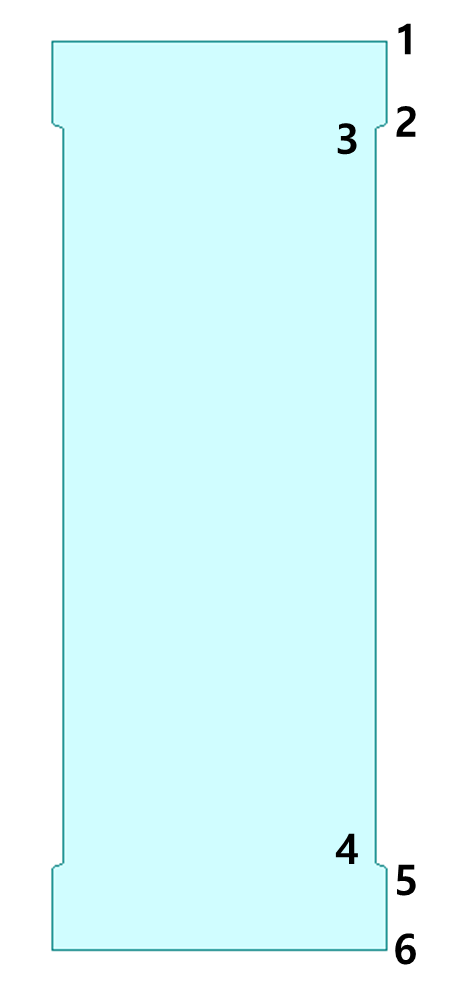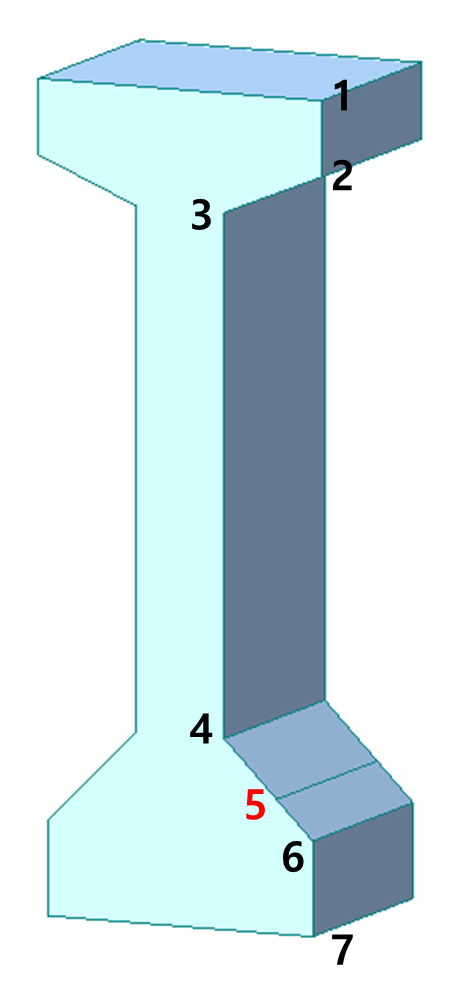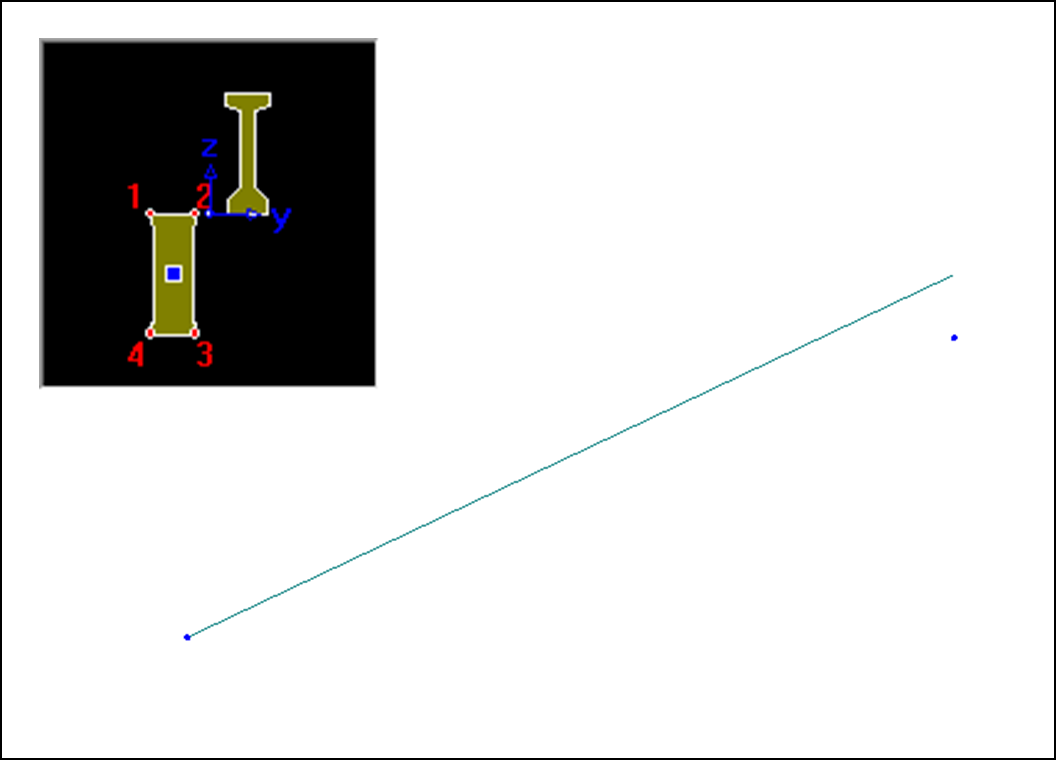Beginning section (6 Nodes) End section (7 Nodes) Tapered Section result

Sometimes, due to user's mistake, unnecessary points (such as point #5 in the end cross-section in the image above) may be added when creating a cross-section in SPC. In such cases, the Tapered Section may appear to be well-created in the Section Data, but it will not be correctly applied to the Element due to the difference in the number of points between the beginning and end cross-sections. To resolve this, you need to create a new cross-section without the unnecessary point.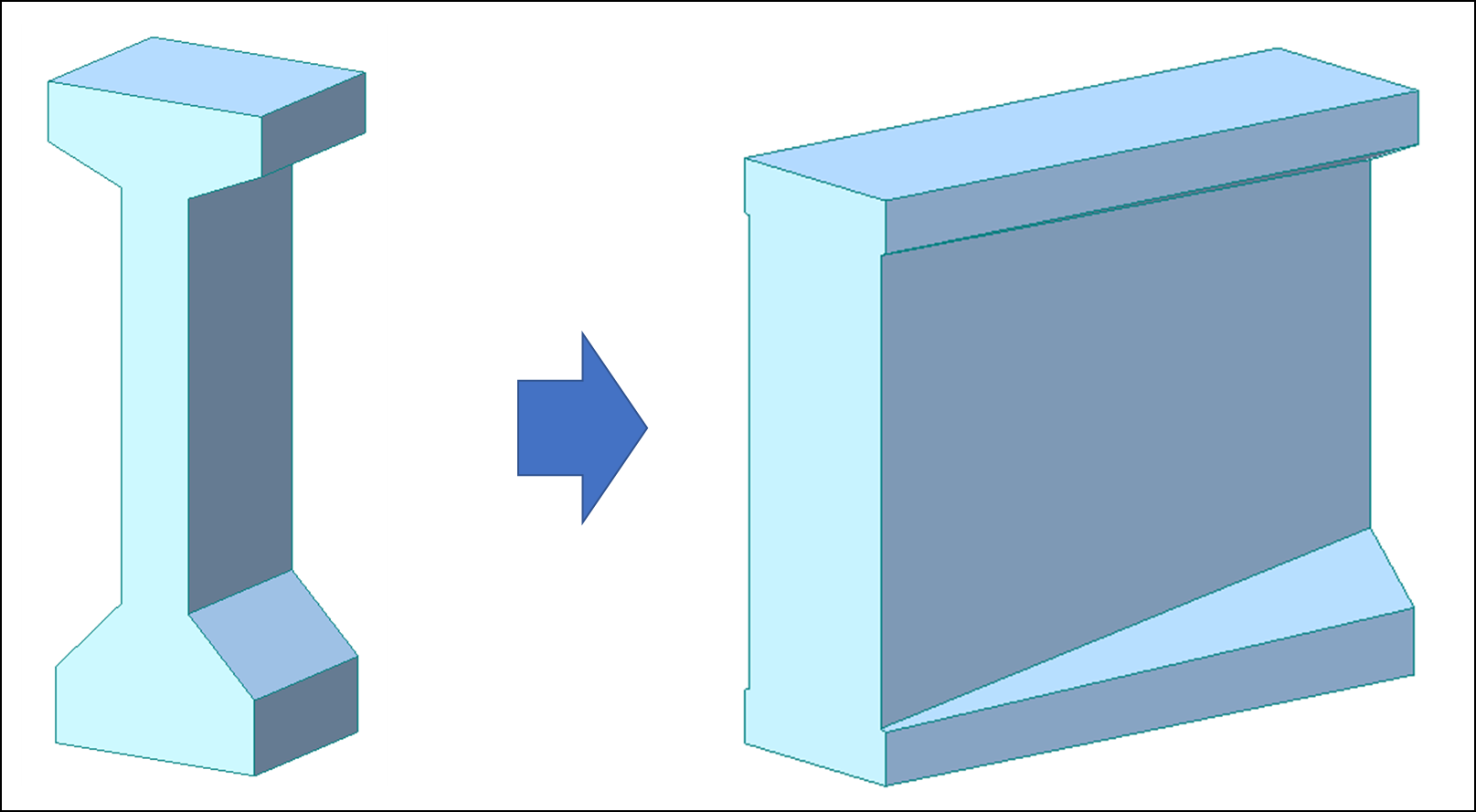Tapered Section result after modifying the end cross-section

Example 2) In case the points in the cross-sections at the beginning and end do not match 1:1(Unequal number of cross-section points)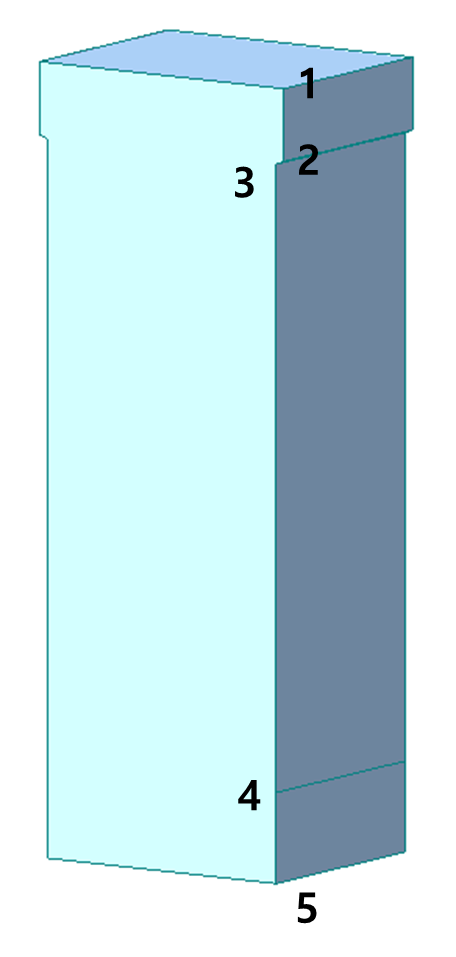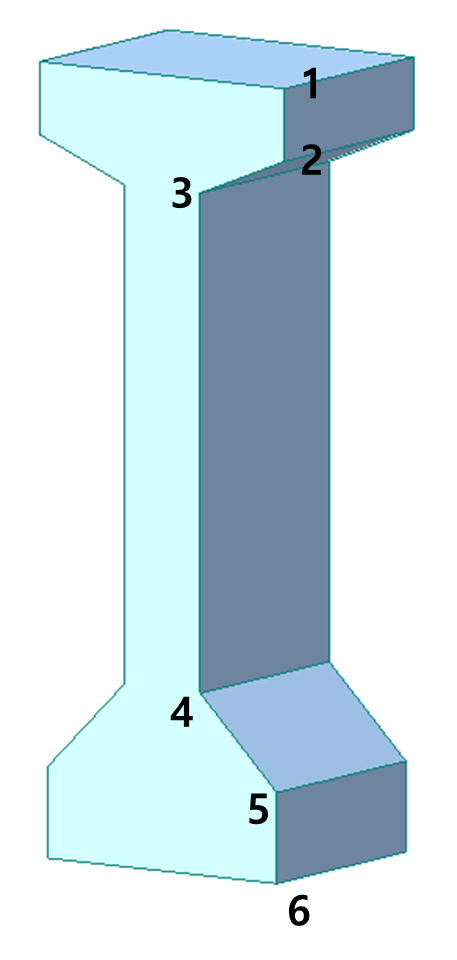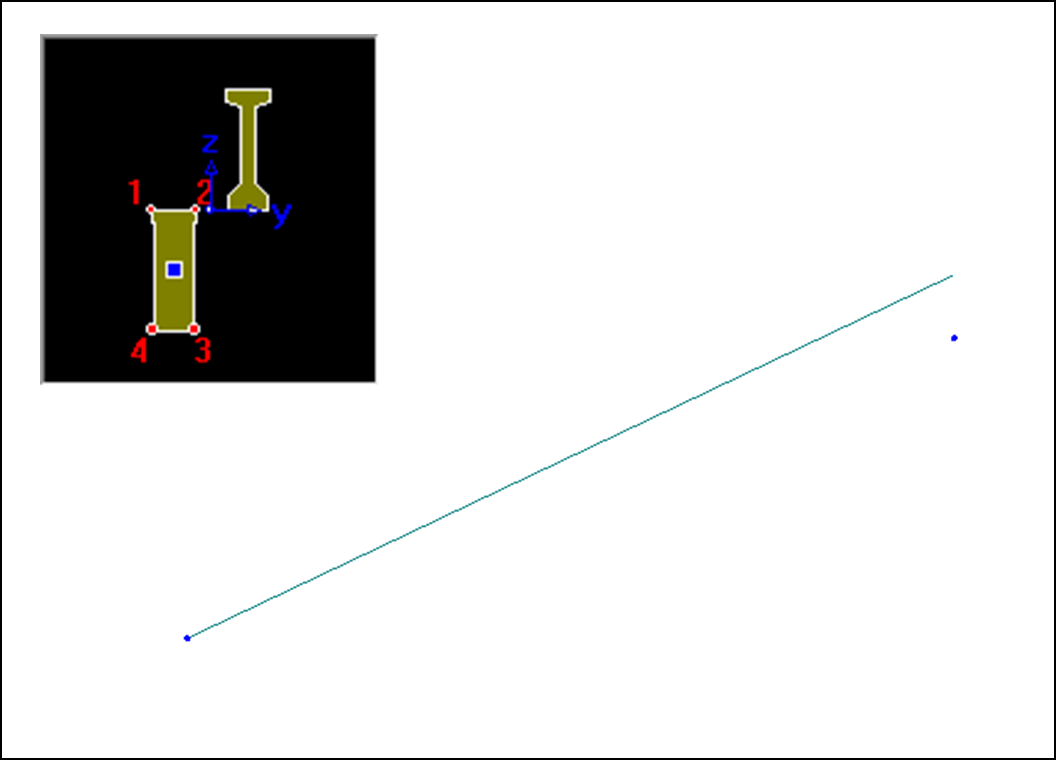Beginning section (5 Points) End ssection (6 Points) Tapered Section result

In case of example 2), unlike example 1), the points in the beginning and end sections do not match 1:1 due to the difference in the original cross-section shapes at the beginning and end, rather than an unnecessary point. In such cases, you need to add arbitrary points to the cross-section to match the number of points in the beginning and end sections.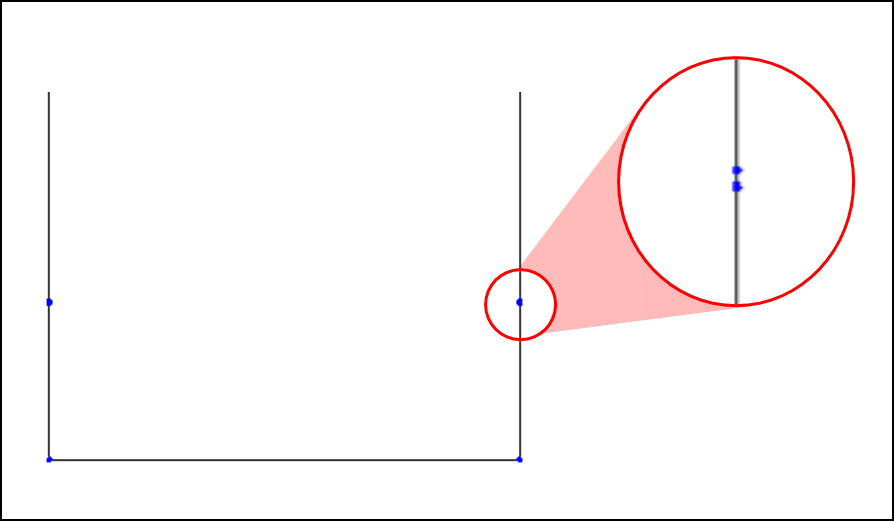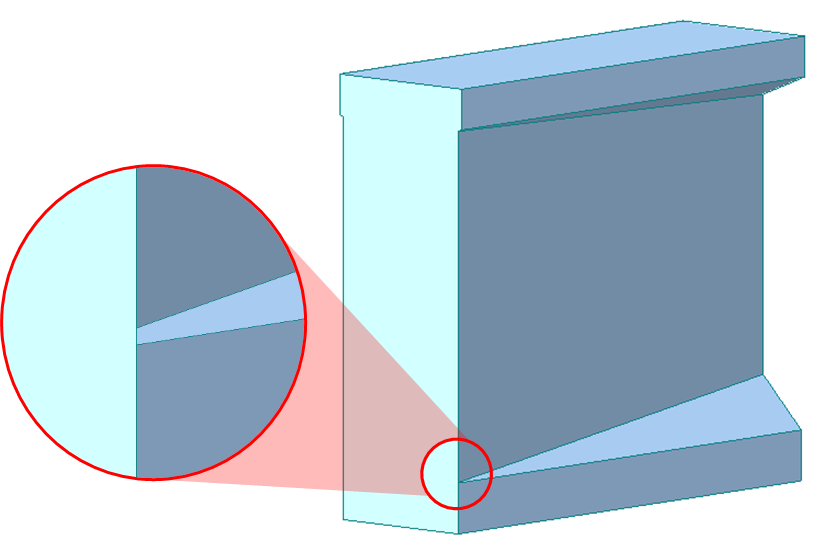Add a point to the beginning cross-section (at position 4) Tapered Section result

## Question 2

The shape of the Element with a Tapered Section is incorrect.
- The points in the beginning and end cross-sections are not properly matched.

Check if the order of points is the same when creating a cross-section in SPC.

When creating a cross-section in SPC, it is common to import a DXF file that was created in CAD, in addition to drawing it directly. When doing this, it is important to remember the point order that was created in CAD to generate the cross-section. In midas Civil, you should note that the points in the tapered section are matched 1:1, taking into account the point order of both the beginning and end cross-sections. Therefore, if the point creating order of the beginning and end cross-sections is different, the shape of the section may be distorted, as shown in the image below.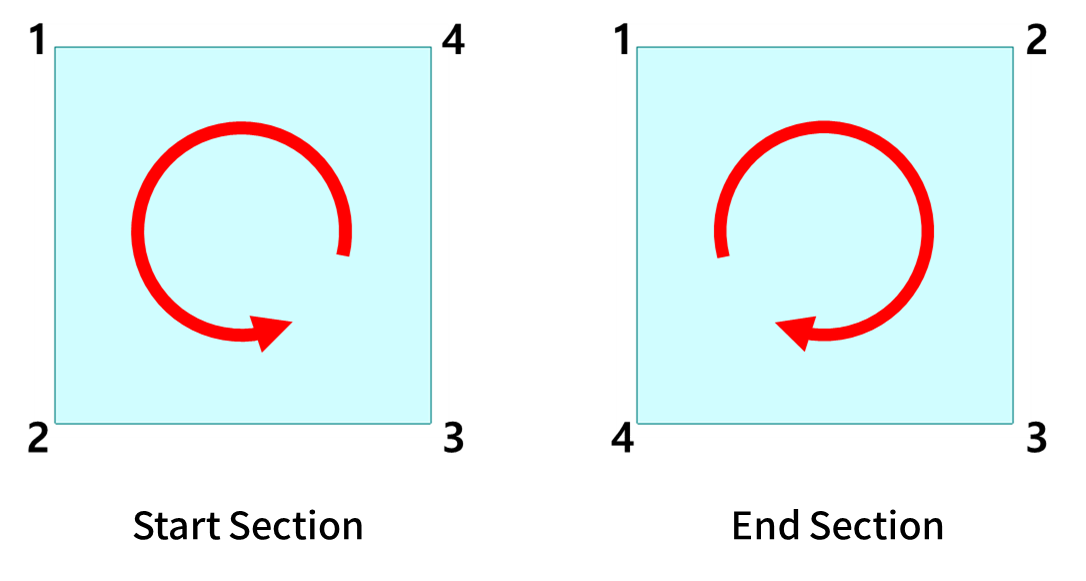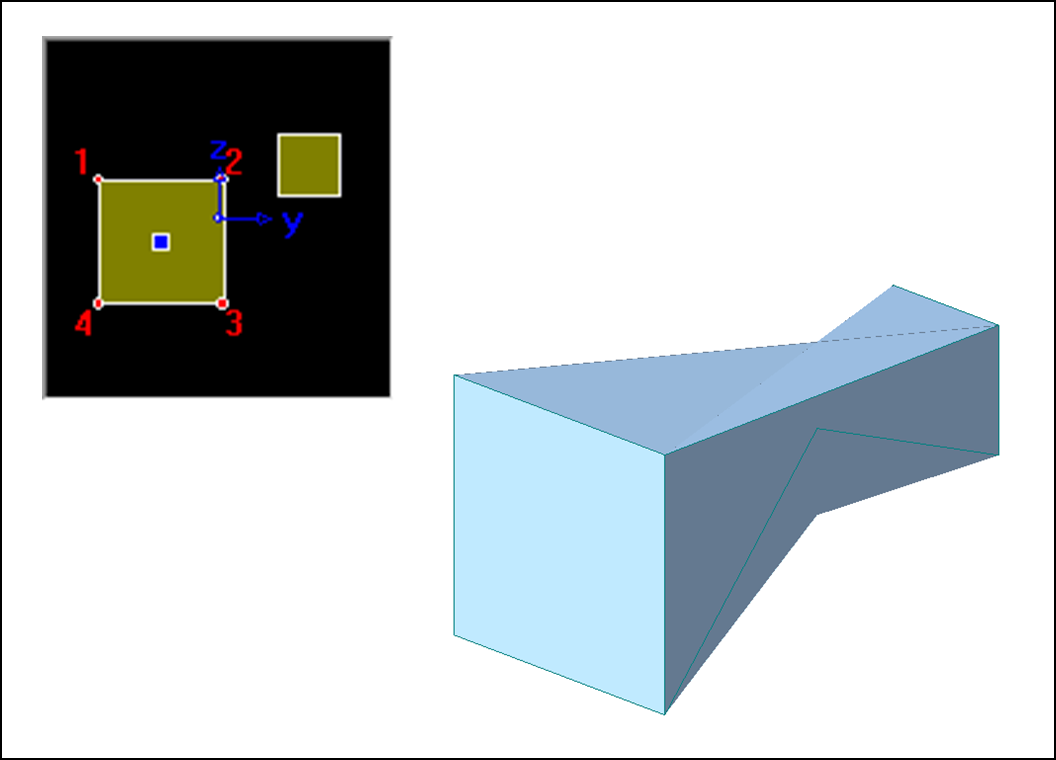The point order of the beginning and end section Tapered Section result

Therefore, if the shape is distorted when applying Tapered Section, you need to consider the Point creating order of the beginning and end sections and create a new section accordingly.

(If you used a dxf file drawn in CAD to create the section in SPC, you will need to redraw it in CAD again because it is not possible to modify the point order of the section in SPC.)

0